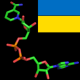# Day 5: Metaoperators

In the Day 4 box, we saw an interesting implementation for the factorial function:

```    sub fac(Int \$n) {
[*] 1..\$n
}
```

Okay, so how does that work? Opening up today’s Advent box provides some answers!

Perl 6 has a number of different “meta operators” that modify the existing operators to perform more powerful functions.

The square brackets about are an example of the “reduce metaoperator”; they cause an infix operator to become a list operator that acts as though the infix was placed between each pair of elements. For example, the expression

```    [+]  1, \$a, 5, \$b
```

is essentially the same as writing

```    1 + \$a + 5 + \$b
```

This gives us a handy mechanism to “sum all elements in a list”:

```    \$sum = [+] @a;            # sum all elements of @a
```

Most of the infix operators (including user-defined operators) can be placed inside of square brackets to turn them into reductions:

```    \$prod = [*] @a;           # multiply all elements of @a

\$mean = ([+] @a) / @a;    # calculate mean of @a

\$sorted = [<=] @a;        # true if elements of @a are numerically sorted

\$min = [min] @a, @b;      # find the smallest element of @a and @b combined
```

So, in the factorial subroutine above, the expression `[*] 1..\$n` returns the product of multiplying all of 1 through \$n together.

Another useful metaoperator is the “hyper” operator. Placing `»`
and/or `«` (or the ASCII `>>` and `<<` equivalents) next to an operator makes it “hyper”, which causes it operate on elements of lists. For example, the following calculates `@c` as the pairwise addition of the elements in `@a` and `@b`:

```    @c = @a »+« @b;
```

In Perl 5, we’d generally write something like

```    for (\$i = 0; \$i < @a; \$i++) {
\$c[\$i] = \$a[\$i] + \$b[\$i];
}
```

which is quite a bit longer.

As with the square brackets above, we can use hyper on a variety of operators, including user-defined operators:

```    # increment all elements of @xyz
@xyz»++

# each element of @x is the smaller of @a and @b
@x = @a »min« @b;
```

We can also flip the angles to enable a scalar to act like an array:

```    # multiply each element of @a by 3.5
@b = @a »*» 3.5;

# multiply each element of @x by \$m and add \$b
@y = @x »*» \$m »+» \$b;

# invert all elements of @x
@inv = 1 «/« @x;

# concatenate @last, @first to produce @full
@full = (@last »~» ', ') »~« @first;
```

Of course, reductions and hyper operators can be combined in expressions:

```    # calculate the sum of squares of @x
\$sumsq = [+] ( @x »**» 2);
```

There are many other metaoperators available, including `X` (cross), `R` (reverse), `S` (sequential). In fact, the “in-place” operators such as `+=`, `*=`, `~=`, are just meta forms created by suffixing an operator with an equals sign:

```    \$a += 5;      # same as \$a = \$a + 5;
\$b //= 7;     # same as \$b = \$b // 7;
\$c min= \$d;   # same as \$c = \$c min \$d;
```

## 15 thoughts on “Day 5: Metaoperators”

1.ragnar says:

\$c min= \$d; is one of the most awesome things i’ve seen since … i last learned something new about perl6!
thank you

2.bened says:

Wow, this is very impressive! Can’t wait to use this in real world applications!

3.RonW says:

Very impressive, but I can’t help thinking about “APL”. Will we soon need special keyboards to code in Perl?

(And yes, APL did have bi- and tri-graphs for all its special characters, but that made for a lot of extra typing)

4.jmo says:

Wow, just like APL but 50 years later!

in APL direct definition:

fac: */ιω

where ω is a the argument of the “fac” function, and “/” is the reduction operator. As for the “hyper” operator, this was the default operator for scalar functions in APL – it was never explicit. You could write “A + B” and if A and B were conformal arrays, the result was a conformal array where each element was the result of adding each corresponding element of A and B.

5.John B says:

The influence of LISP continues… “meta operators”

6.Deriksims says:

Да уж. По поводу коментариев – навеяла на меня где-то услышанная фраза:
Только наш клиент в графе “Телефон” мог написать Samsung.

7.Игорь says:

Дискутировать можно бесконечно, поэтому просто поблагодарю автора. Спасибо!

8.Christopher Bottoms says:

Wonderful article! As far as I can tell, just one line of code needed an update (a “flat”) for it to work this Christmas:

```\$min = [min] flat @a, @b;
```

Thanks so much for your years of work on Perl 6! Merry Christmas!

This site uses Akismet to reduce spam. Learn how your comment data is processed.